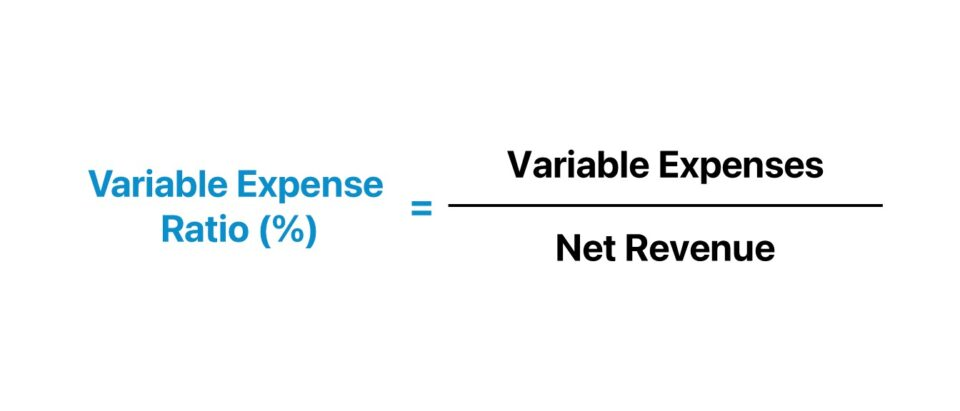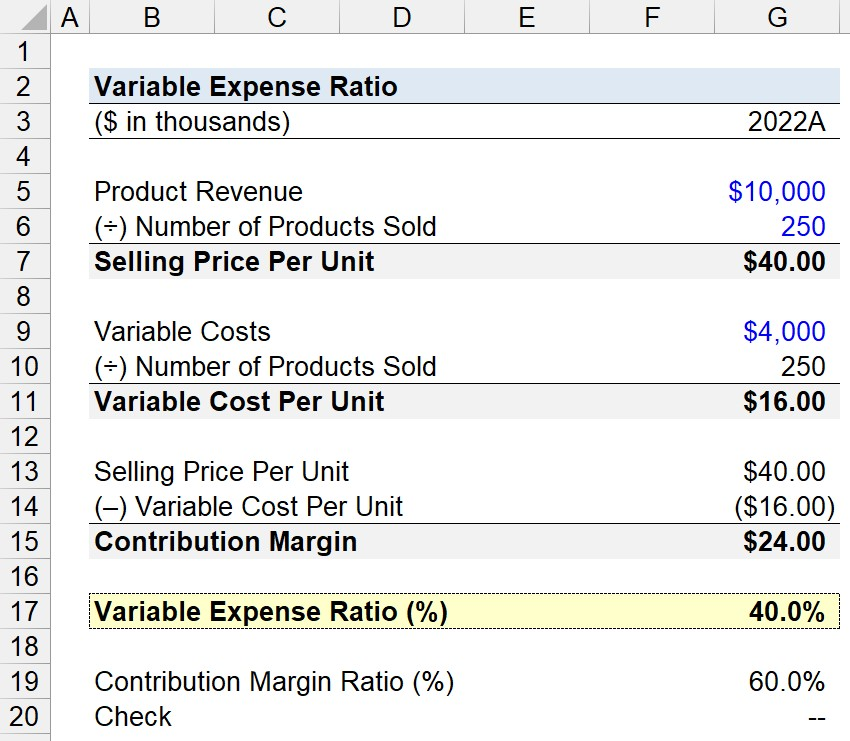Welcome to Wall Street Prep! Use code at checkout for 15% off.# Variable Expense Ratio

Guide to Understanding the Variable Expense Ratio## How to Calculate Variable Expense Ratio?

The variable expense ratio, or “variable cost ratio,” measures the proportion of a company’s cost structure composed of variable costs.

Since the variable expense ratio expresses variable costs as a percentage of net sales, the metric is useful for analyzing the break-even point (BEP) and performing cost-volume-profit analysis (CVP).

Based on the insights derived from the metric, a company’s management team can set pricing rates and production scheduling appropriately to maximize its profit margins.

Therefore, the variable expense ratio measures the amount a company must pay in variable costs to produce a specific number of product units, relative to the revenue generated from selling those products.

The expenses incurred by companies can be classified as either: 1) fixed expenses or 2) variable expenses.

• Fixed Expense → A fixed expense does not fluctuate based on production volume or sales. For instance, the rental cost of a manufacturing facility is fixed, because the amount remains constant.
• Variable Expense → In contrast, a variable expense is tied to the production level and sales volume of a company. The total expense incurred increases amid a period of higher production, and vice versa. For example, the cost of direct materials and commissions are examples of variable costs.

The variable expense ratio is equal to the total variable expenses of a company divided by its total net sales, expressed in percentage form.

• High Variable Cost Ratio → High Contribution Margin (More Profitable)
• Low Variable Cost Ratio → Low Contribution Margin (Less Profitable)

## Variable Expense Ratio Formula

The formula to calculate the variable expense ratio is as follows.

Variable Expense Ratio = Variable Expenses ÷ Net Sales

To convert the output into percentage form, simply multiply by 100.

Conversely, the variable expense ratio can be calculated using the contribution margin.

Variable Expense Ratio = 1 Contribution Margin

The contribution margin is the difference between net sales and total variable costs, expressed as a percentage, to quantify the amount of revenue remaining that can be allocated toward fixed costs.

## Variable Expense Ratio Calculator

We’ll now move to a modeling exercise, which you can access by filling out the form below.#### Excel TemplateSubmitting...

## Variable Expense Ratio Calculation Example

Suppose we’re tasked with calculating the variable expense ratio of a company that generated \$10 million in product revenue in fiscal year 2022.

The total number of products sold was 250k units, while the variable costs were \$4 million.

• Product Revenue = \$10 million
• Number of Products Sold = 250k
• Variable Costs = \$4 million

By dividing the product revenue by the number of products sold, the selling price per unit is calculated to be \$40.

If we repeat the same step, but switch out product revenue with variable costs, the variable cost per unit is \$16.

• Selling Price Per Unit = \$10 million ÷ 250k = \$40.00
• Variable Cost Per Unit = \$4 million ÷ 250k = \$16.00

The variable expense ratio is 40%, which we determined by dividing the selling price per unit by the variable cost per unit.

• Variable Expense Ratio (%) = \$16.00 ÷ \$40.00 = 40.0%

In conclusion, we can check our work using the alternative formula with the contribution margin, which yields a variable expense ratio of 40.0%.

• Variable Expense Ratio (%) = 1 – 60.0% = 40.0%Step-by-Step Online Course

### Everything You Need To Master Financial Modeling

Enroll in The Premium Package: Learn Financial Statement Modeling, DCF, M&A, LBO and Comps. The same training program used at top investment banks.